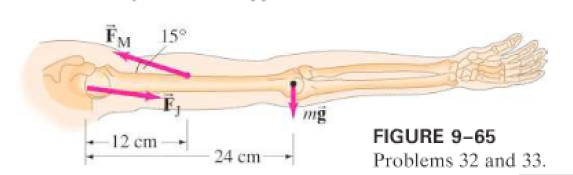×
Get Full Access to Physics: Principles With Applications - 6 Edition - Chapter 9 - Problem 32p
Get Full Access to Physics: Principles With Applications - 6 Edition - Chapter 9 - Problem 32p

×

# (II) (a) Calculate the magnitude of the force, FM requiredISBN: 9780130606204 3

## Solution for problem 32P Chapter 9

Physics: Principles with Applications | 6th Edition

• Textbook Solutions
• 2901 Step-by-step solutions solved by professors and subject experts
• Get 24/7 help from StudySoup virtual teaching assistantsPhysics: Principles with Applications | 6th Edition

4 5 1 310 Reviews
28
2
Problem 32P

(II) (a) Calculate the force, $$F_{M}$$, required of the "deltoid" muscle to hold up the outstretched arm shown in Fig.. The total mass of the arm is. (b) Calculate the magnitude of the force $$F_{J}$$ exerted by the shoulder joint on the upper arm.Equation Transcription:Text Transcription:

F_{M}

F_{J}

Step-by-Step Solution:

Step-by-step solution

Step 1 of 5 ^

We are going to draw the free body diagram of the systemShoulder joint is taken as pivot point O

Fm; The force exerted by muscle.

W: the weight of the arm/

Fj; the reaction force exerted from the joint.

dfm; the force  distance from muscle force to pivot pint

dw; the force distance from weight of the arm to pivot point.

Step 1 of 5 ^

We are going write the second condition of equilibrium as taking  the torques around pivot point to find the force exerted by muscle.The force exerted by muscle to hold the arm.

Step 2 of 5

Step 3 of 5

## Discover and learn what students are asking

Calculus: Early Transcendental Functions : Conservative Vector Fields and Independence of Path
?In Exercises 5 - 10, determine whether the vector field is conservative. $$\mathbf{F}(x, y)=15 x^{2} y^{2} \mathbf{i}+10 x^{3} y \mathbf{j}$$

Statistics: Informed Decisions Using Data : Testing the Significance of the Least-Squares Regression Model
?In Problems 5–10, use the results of Problems 7–12, respectively, from Section 4.2 to answer the following questions: (a) What are the estima

Unlock Textbook Solution